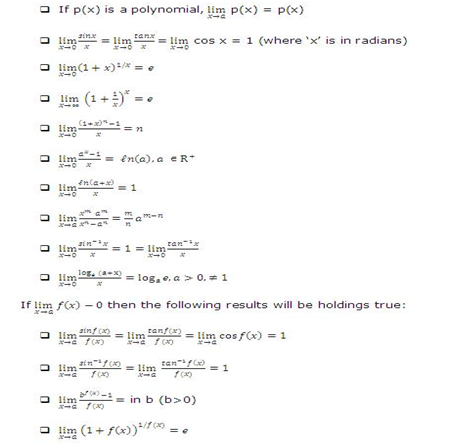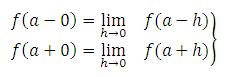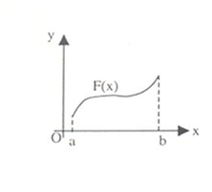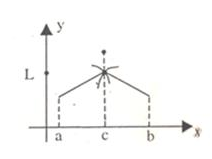Click to Chat

1800-1023-196

+91-120-4616500

CART 0

• 0

MY CART (5)

Use Coupon: CART20 and get 20% off on all online Study Material

ITEM
DETAILS
MRP
DISCOUNT
FINAL PRICE
Total Price: Rs.

There are no items in this cart.
Continue Shopping• Complete JEE Main/Advanced Course and Test Series
• OFFERED PRICE: Rs. 15,900
• View Details

```Revision Notes on Limits, Continuity & Differentiability

A. Limits

Limit of a function may be a finite or an infinite number.

If limx→a f(x) = ∞, it just implies that the function f(x) tends to assume extremely large positive values in the vicinity of x = a i.e. limx→0 1/|x|= ∞.

A function is said to be indeterminate at any point if it acquires one of the following values at that particular point

0/0, 0 × ∞, ∞/∞, ∞-∞, 00, 1∞, ∞0.

The 0/0 form is the standard indeterminate form.

The point ‘∞’ cannot be plotted on the paper. It is just a symbol and not a number.

Infinity (∞) does not obey the laws of elementary algebra.

1. ∞ + ∞ = ∞

2. ∞ x ∞ = ∞

3. (a/∞) = 0, if a is finite

4. (a/0) is not defined if a ≠ 0.

5. ab = 0 iff either a = 0 or b = 0 and both ‘a’ and ‘b’ are finite.

In case of limits, it is important to note that the function cannot be manipulated and cancelled as in usual algebra.

For example: (x2-a2)/(x-a) = (x+a)(x-a)/((x-a)) = (x+a)

This can be done in general, but in limits this is not possible until and unless (x-a) ≠ 0 or x ≠ a.

The limit may exist at a point x = a even if the function is not defined at that point.

If a function f is defined at a point ‘a’ i.e. f(a) exists even then it is not necessary that the limit at ‘a’ should exist. Moreover, even if the limit exists it need not be equal to f(a).

Fundamental Results on Limits:

Suppose limx→a f(x) = α and limx→a g(x) = β then we can define the following rules:The above rules are applicable only when both the limits i.e. lim f(x) and g(x) exist separately. In addition to above rules, we have two more rules:

limx→a k f(x) = k limx→a f(x), where k is a constant

limx→a f [g(x)] = f [limx→a g(x)] = f(m), provided f is continuous at g(x) = m.

Some standard limits which should be remembered include:Following are some of the frequently used series expansions:Let f(x) be a function defined on domain D and let ‘a’ be a point such that every neighborhood of ‘a’ contains infinitely many points of D. A real number ‘l’ is called the left hand limit of f(x) at x = a iff for every ∈ > 0 there exists a δ > 0 such that a – δ < x < a ⇒ | f(x) – l| < ∈.

In such a case we write limx→a- f(x) = l.

Thus, limx→a- f(x) = l if f(x) tends to l as x tends to a from the left hand side.

Similarly, a real number ‘l’ is said to be a right hand limit of f(x) at x = a iff for every ∈ > 0, there exists a δ > 0 such that a < x < a + δ ⇒ | f(x) – l | < ∈ and we write it as limx→a+ f(x) = l*.

Hence, l* is said to be the right hand limit of f(x) at x =a iff f(x) tends to l* as x tends to ‘a’ from the right hand side.

Let the function f(x) be defined in x ∈ [a, b]. At times, we might have to calculate lim x→b f(x) or lim x→a f(x).

In such a case, lim x→a f(x) = x→a+ f(x) = R.H.L at x = a, as there is no left neighborhood of x = a.

Similarly, lim x→b f(x) or lim x→b- f(x) = L.H.L at x = b as there is no left neighborhood of x = b.

Eg: f(x) = cos-1 x

lim x→1 f(x) = lim x→1- cos-1 x = 0.

lim x→-1 f(x) = lim x→-1+ cos-1 x = π.

The term infinite limit means that when x tends to a particular value 'a', then the limit of the function tends to infinity i.e.limx→2 f(x) = ∞.

In questions which involve the evaluation of limit at infinity, the function f(x) should first be changed to g(1/x) and then we can evaluate the value at ∞.

If while calculating limits, infinite limit is encountered i.e. a zero is obtained in the denominator as x→a, then there can be two cases:

1. The term (x-a) gets cancelled from the numerator and denominator both.

2. If it does not get cancelled, then the value of the limit is put as infinity.

3. Such limits are termed as improper limits i.e. limx→∞ 1/x2 =∞.

Let f(x), g(x) and h(x) be there real numbers having a common domain D such that h (x) ≤ f(x) ≤ g(x) ∀ x ∈ D. If limx→a h(x) = limx→a g(x)= l, then limx→a f(x) = l. This is known as Sandwich Theorem.

Let f(x) and g(x) be functions differentiable in the neighborhood of the point a, except may be at the point a itself. If  limx→a f(x) = 0 = limx→a g(x) or limx→af(x) = ∞ = limx→ag(x), then

limx→a f(x)/g(x) = limx→a f' (x)/g(x) = limx→a f' (x)/g'(x) provided that the limit on the right either exists as a finite number or is ± ∞ .

The concept of limits and continuity is quite interrelated. Limits form the base for continuity.

B. Left and Right Limit

Let y = f(x) be a given function, and x = a is the point under consideration. Left tendency of f(x) at x = a is called its left limit and right tendency is called its right limit.

Left tendency (left limit) is denoted by f(a - 0) or f(a-) and right tendency (right limit) is denoted by f(a + 0) or f(a+) and are written aswhere 'h' is a small positive number.

Thus for the existence of the limit of f(x) at x = a, it is necessary and sufficient that f(a – 0)  = f(a + 0), if these are finite or f(a-0) and f(a+0) both should be either +∞ or -∞.

C. Evaluation of Algebraic Limits

It is quite possible for a function to have infinite limit as x → a. If limx→a f(x) = ∞, it would simply mean that function has tendency to assume very large positive values in the neighborhood of x = a, for example limx→0 1/|x| = ∞.

Some of the methods of evaluating limits include:

1. Direct Substitution Method:

Consider the following limits (i) limx→a f(x) and (ii) limx→a φ(x)/Ψ(x).

If f(a) and φ(a)/Ψ(a) exist and are fixed real numbers and Ψ(a) ≠ 0, then we say that limx→a f(x) = f(a) and limx→a  φ(x)/Ψ(x) =  φ(a)/Ψ(a).

?2. Factorization Method

Consider limx→a f(x)/g(x). If by substituting x = a, f(x)/g(x) reduces to the form 0/0, then (x-a) is a factor of both f(x) and g(x). So, we first factorize f(x) and g(x) and then cancel out the common factor to evaluate the limit.

3. Rationalization Method:

This method is generally used in cases where either the numerator or the denominator or both involve expression consists of square roots and on substituting the value of x, the rational expression takes the form 0/0, ∞/∞.

4. Evaluation of Algebraic limits at infinity:

We know that lim x→∞ 1/x = 0 and lim x→∞ 1/x2 = 0.

Then lim x→∞ f(x) = lim y→0 f(1/y).

Note:

When infinite limit is encountered i.e. '0' in the denominator as x→a, then either (x-a) get cancelled from numerator and denominator both and if it is not possible then the limit's value is put as infinity. Such limits are called   improper limits i.e. limx→∞ 1/x2 = ∞.

D. Limits using Sandwich Theorem

Let f(x), g(x) and h(x) be three real numbers having a common domain D such that h(x) ≤ f(x) ≤ g(x) ∀ x ∈ D. If limx→a h(x) = limx→a g(x) = l, then limx→a f(x) = l. This is known as Sandwich Theorem.

E. L Hospital’s Rule

The L Hospital’s rule is used in cases where the limit obtained is in the form of 0/0, ∞/∞, ∞ – ∞,0, ∞, 00, ∞0, 1∞. These are known as indeterminate forms. According to this rule,

Let f(x) and g(x) be functions differentiable in the neighborhood of the point a, except may be at the point a itself. If lim x→a f(x) = 0 = limx→a g(x) or limx→af(x) = ∞ = limx→a g(x), then

limx→a f(x)/g(x) = limx→a f ’(x)/g'(x) provided that the limit on the right either exists as a finite number or is ± ∞ .

F. Continuity

A function f(x) is said to be continuous at x= a if

limx→a- f(x)= limx→a+ f(x)=f(a)

Thus, unlike limits, for continuity it is essential for the function to be defined at that particular point and the limiting value of the function should be equal to f(a).

The function f(x) will be discontinuous at x = a in either of the following situations:

limx→a- f(x) and limx→a+ f(x) exist but are not equal.

limx→a- f(x) and limx→a+ f(x) exist and are equal but not equal to f(a).

f(a) is not defined.

At least one of the limits does not exist.

If you are required to comment on the continuity of a function, then you may just look for the points on the domain where the function is not defined.

Some important properties of continuous functions:

If the functions f(x) and g(x) are both continuous at x = a then the following results hold true:

1. cf (x) is continuous at x = a where c is any constant.

2. f(x) + g(x) is continuous at x = a.

3. f(x).g(x) is continuous at x= a.

4. f(x)/g(x) is continuous at x= a, provided g(a) ≠ 0.

If a function f is continuous in (a, b), it means it is continuous at every point of (a, b).

If f is continuous in [a, b] then in addition to being continuous ay every point of domain, f should also be continuous at the end points i.e. f(x) is said to be continuous in the closed interval [a, b] if

1. f(x) is continuous in (a, b)

2. limx→a+ f(x) = f(a)

3. limx→a- f(x) = f(a)While solving problems on continuity, one need not calculate continuity at every point, in fact the elementary knowledge of the function should be used to search the points of discontinuity.

In questions like this where a function h is defined as

h(x) = f(x) for a < x < b

g(x) for b < x < c

The functions f and g are continuous in their respective intervals, then the continuity of function h should be checked only at the point x = b as this is the only possible point of discontinuity.

If the point ‘a’ is finite, then the necessary and sufficient condition for the function f to be continuous at a is that limx→a- f(x) and limx→a+ f(x) should exist and be equal to f(a).

A function continuous on a closed interval [a, b] is necessarily bounded if both a and b are finite. This is not true in case of open interval.

If the function u = f(x) is continuous at the point x = a, and the function y = g(u) is continuous at the point u = f(a), then the composite function y = (gof)(x) = g(f(x)) is continuous at the point x = a.

Given below is the table of some common functions along with the intervals in which they are continuous:

Functions f(x)

Interval in which f(x) is continuous

Constant C

(-∞,∞)

bn, n is an integer > 0

(-∞,∞)

|x-a|

(-∞,∞)

x-n, n is a positive integer.

(-∞,∞) – {0}

a0xn + a1xn-1 +........ + an-1x + an

(-∞,∞)

p(x)/q(x), p(x) and q(x) are polynomials in x

R - {x:q(x) = 0}

sin x

R

cos x

R

tan x

R-{nπ:n = 0,±1,........}

cot x

R-{(2n-1)π/2: n = 0,±1,± 2,........}

sec x

R-{(2n-1)π/2: n = 0,±1,± 2,........}

ex

R

ln x

(0, ∞)

If you know the graph of a function, it can be easily judged without even solving whether a function is continuous or not. The graph below clearly shows that the function is discontinuous.If limx→a- f(x) = L1 and limx→a+ f(x) = L2, where L1 and L2 are both finite numbers then it is called discontinuity of first kind or ordinary discontinuity.

A function is said to have discontinuity of second kind if neither limx→a+ f(x) nor limx→a- f(x) exist.

If any one of limx→a+ f(x) or limx→a- f(x) exists and the other does not then the function f is said to have mixed discontinuity.

If limx→a f(x) exists but is not equal to f(a), then f(x) has removable discontinuity at x = a and it can be removed by redefining f(x) at x = a.

If limx→a f(x) does not exist, then we can remove this discontinuity so that it becomes a non-removable or essential discontinuity.

A function f(x) is said to have a jump discontinuity at a point x = a if, limx→a- f(x) ≠ limx→a+ f(x) and f(x) and may be equal to either of previous limits.

The concepts of limit and continuity are closely related. Whether a function is continuous or not can be determined by the limit of the function.

A function f(x) is said to be differentiable at point P iff there exists a unique tangent at point P.

A function f(x) is differentiable at a point P iff the curve does not have P as a corner point i .e. the curve is not differentiable at the points where there are holes or sharp edges.

The function f(x) is said to be differentiable in interval (a, b) if f(x) is differentiable at every point of interval (a, b).

The function f(x) is said to be differentiable in closed interval [a, b] if in addition to being differentiable in (a, b), f(x) is differentiable at x = a from the right and at x = b from the left.

A function which is differentiable at x = a is necessarily continuous at x = a but not vice versa.

The sum, difference, product and quotient of two differentiable functions is differentiable.

The composition of differentiable functions is a differentiable function.

Continuity and differentiability of functions:

The identity, exponential and logarithmic functions are continuous and differentiable in their domain.

The greatest integer function, least integer function and the fractional part functions are continuous and differentiable at all points except at integral values.

The signum function is continuous and differentiable at all points except at x = 0.

The polynomial function is continuous and differentiable everywhere.

The trigonometric functions i.e. sine, cosine, tangent, cotangent, secant and cosecant are continuous and differentiable in their domain.

The inverse trigonometric functions are continuous and differentiable in their domain.

```### Course Features

• 728 Video Lectures
• Revision Notes
• Previous Year Papers
• Mind Map
• Study Planner
• NCERT Solutions
• Discussion Forum
• Test paper with Video Solution